Engineering Jobs   »   CIVIL ENGINEERING QUIZ

# DFCCIL’21 CE: Daily Practice Quiz. 31-July-2021

Quiz: Civil Engineering
Exam: DFCCIL-JE
Topic: STRENGTH OF MATERIAL

Each question carries 1 mark
Negative marking: 1/4 mark
Time: 8 Minutes

Q1. Which formula CORRECTLY depicts the elongation in a composite body?
(a) δ=P/E [l_1/A_1 +l_2/A_2 +l_3/A_3 +l_4/A_4 +⋯.]
(b) δ=P/AE [l_1+l_2+l_3+l_4….]
(c) δ=P/AE [l_1-l_2+l_3-l_4+⋯.]
(d) δ=Pl/E [1/A_1 +1/A_1 +1/A_1 +1/A_1 +⋯]

Q2. What will be the thermal stress developed in a rod having a diameter of 4 cm and length of 2m. it experiences heating from temperature 50°C to 200°C. the coefficient of thermal expansion is:
α=10×10^(-6) \/°C and young modulus is 250 GPa
(a) 300
(b) 325
(c) 350
(d) 375

Q3. A rod with a length of 100 cm and diameter of 4 cm undergoes and axial pull of 50 kN. What is the stress (in N/mm²)?
(a) 0.04
(b) 0.4
(c) 4
(d) 40

Q4. Determine the value of shear force at point ‘A’ in the figure shown below: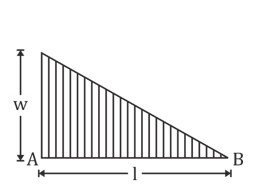(a) (wl^2)/6
(b) (wl^2)/2
(c) wl^2
(d) (wl^2)/3

Q5. The diagram shown below shows the shearing force diagram for a beam.
Which of the following is the correct bending moment diagram for the above shear force diagram?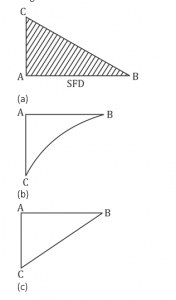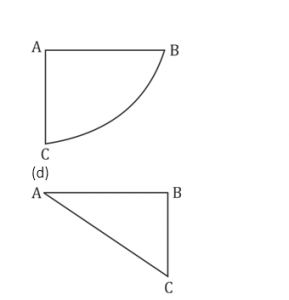Q6. Maximum principal stress theory was postulated by __________.
(a) St. Venant
(b) Mohr
(c) Rankine
(d) Tresca

SOLUTION
S1. Ans.(a)
Sol. Bar is composite so axial force is same throughout the bars.Total elongation is the sum of each bars elongation.
Total elongation δ=δ_1+ δ_2+δ_3+⋯
δ=(Pl_1)/(A_1 E)+(Pl_2)/(A_2 E)+(Pl_3)/(A_3 E)……
δ=P/E [l_1/A_1 +l_2/A_2 +l_3/A_3 ]

S2. Ans.(d)
Sol. Thermal stress in rod
σ_thermal=α∆TE
=10×10^(-6)×(200-50)×250×10^9
=375 MPa

S3. Ans.(d)
Sol. Given,
L = 100 cm, d = 4 cm, P = 50 kN
σ=P/Q=P/(π/4 d^2 )
=4P/(πd^2 ) =(4×50×10^3)/(π×[0.04]^2 )
=39.789 MPa
=40 N\/mm^2
S4. Ans.(a)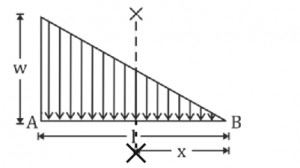f=∫_0^L▒〖W_(x-x) dx〗
=∫_0^L▒〖wx/l.dx〗
=[(wx^2)/2L]_0^l
=wL/2 – it is at L/3 from A then bending moment at A
M_A=f.x
=WL/2.L/3
=(WL^2)/6

S5. Ans.(a)
Sol. In SFD value of shear force is decreasing from A to B. So, slope of bending moment diagram also has decreasing slope.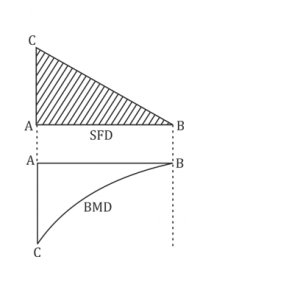S6. Ans.(c)
Sol. maximum principal stress theory also known as Rankine’s theory because it is postulated by Rankine.

Sharing is caring!